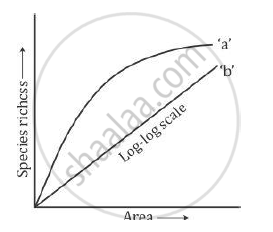# The Following Graph Shows the Species - Area Relationship. Answer the Following Questions as Directed. - Biology

The following graph shows the species - area relationship. Answer the following questions as directed.(a) Name the naturalist who studied the kind of relationship shown in the graph. Write the observations made by him.

(b) Write the situations as discovered by the ecologists when the value of 'Z'
(Slope of the line) lies between.

(i) 0.1 and 0.2

(ii) 0.6 and 1.2

What does 'Z' stand for?

(c) When would the slope of the line 'b' become steeper?

#### Solution

(a) The German naturalist and geographer Alexander von Humboldt studied the species–area relationship. He found that species richness within a region increased with increasing area but up to a certain limit. The relationship between species richness and area turned out to be rectangular hyperbola for a wide variety of taxa.

(b)

(i) If the value of Z lies in the range of 0.1−0.2, then it is regardless of taxonomic group or region.

(ii) If the value of Z lies in the range of 0.6−1.2, then the slope of the line will be much steeper.

Z stands for the slope of the line or regression coefficient.

(c) If the species–area relationship is for very large areas such as the entire continent, then the slope of the line will be much steeper.

Concept: Patterns of Biodiversity
Is there an error in this question or solution?